Next: Low-Energy Scattering Up: Scattering Theory Previous: Determination of Phase-Shifts

# Hard-Sphere Scattering

Let us try out the scheme outlined in the previous section using a particularly simple example. Consider scattering by a hard sphere, for which the potential is infinite for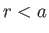, and zero for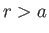. It follows that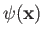is zero in the region, which implies that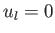for all. Thus,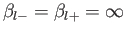(10.103)

for all. Equation (10.97) yields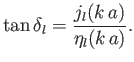(10.104)

In fact, this result is most easily obtained from the obvious requirement that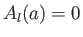. [See Equation (10.95).]

Consider thepartial wave, which is usually referred to as the S-wave. Equation (10.105) gives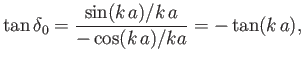(10.105)

where use has been made of Equations (10.60) and (10.61). It follows that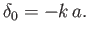(10.106)

The S-wave radial wave function is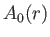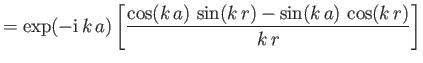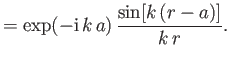(10.107)

[See Equation (10.95).] The corresponding radial wavefunction for the incident wave takes the form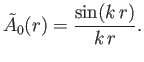(10.108)

[See Equations (10.79), (10.80), (10.94), and (10.107).] It is clear that the actualradial wavefunction is similar to the incidentwavefunction, except that it is phase-shifted by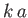.

Let us consider the low- and high-energy asymptotic limits of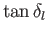. Low energy corresponds to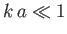. In this limit, the spherical Bessel functions and Neumann functions reduce to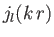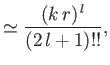(10.109)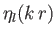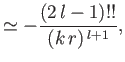(10.110)

where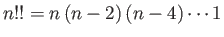. It follows that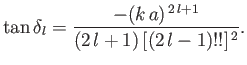(10.111)

It is clear that we can neglect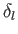, with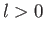, with respect to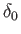. In other words, at low energy, only S-wave scattering (i.e., spherically symmetric scattering) is important. It follows from Equations (10.28), (10.81), and (10.107) that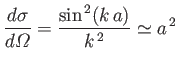(10.112)

for. Note that the total cross-section,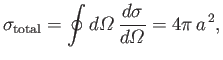(10.113)

is four times the geometric cross-section,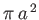(i.e., the cross-section for classical particles bouncing off a hard sphere of radius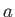). However, low-energy scattering implies relatively long de Broglie wavelengths, so we would not expect to obtain the classical result in this limit.

Consider the high-energy limit,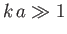. At high energies, by analogy with classical scattering, the scattered particles with the largest angular momenta about the origin have angular momenta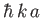(i.e., the product of their incident momenta,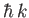, and their maximum possible impact parameters,). Given that particles in theth partial wave have angular momenta, we deduce that all partial waves up to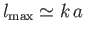contribute significantly to the scattering cross-section. It follows from Equation (10.90) that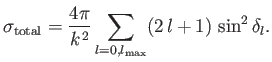(10.114)

Making use of Equation (10.105), as well as the asymptotic expansions (10.62) and (10.63), we find that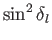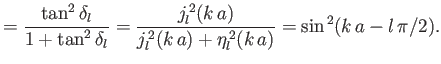(10.115)

In particular,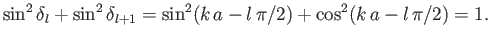(10.116)

Hence, it is a good approximation to write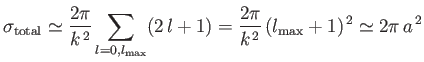(10.117)

. This is twice the classical result, which is somewhat surprising, because we might expect to obtain the classical result in the short-wavelength limit. In fact, for hard-sphere scattering, all incident particles with impact parameters less thanare deflected. However, in order to produce a shadow behind the sphere, there must be scattering in the forward direction (recall the optical theorem) to produce destructive interference with the incident plane wave. The effective cross-section associated with this forward scattering is, which, when combined with the cross-section for classical reflection,, gives the actual cross-section of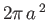.Next: Low-Energy Scattering Up: Scattering Theory Previous: Determination of Phase-Shifts
Richard Fitzpatrick 2016-01-22